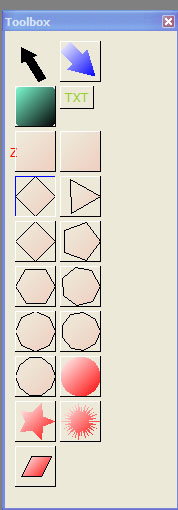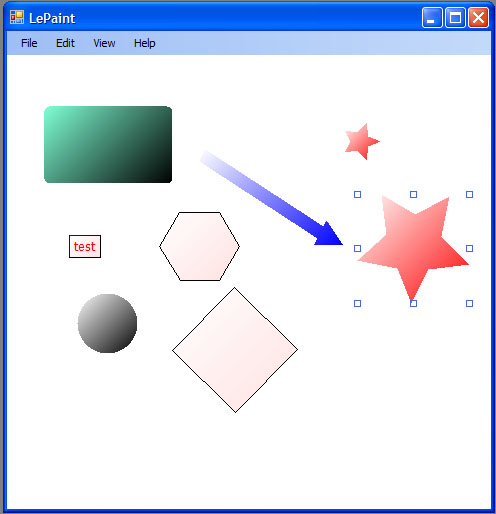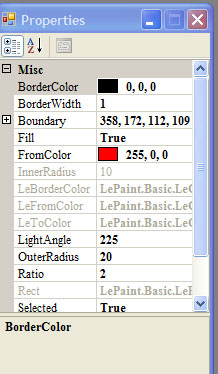## 1

This is a drawing tool I developed in my spare time, it can draw rectangle, circle, and other shapes, you can also move, resize, even rotate some of the shapes. It will be a useful starting point of a complicated graphics project.

## 2

To fully understand this code, the user has to understand some of the C# concepts, reflection, interfaces, inheritance, etc. There is another version of this drawing tool by using WPF, you can find it on Graphics Drawing Tool WPF.aspx.

## 3

This project first creates a tool box which contains all the available drawing tools. Like the following screen capture:Then the user can select these tools to draw on main screen, a screen capture like the following:This project also comes up with a propertybag for user, to dynamically change shape border color, fill color, arrow width, text box content, text size, etc.This project finally provides the user with an option of exporting the drawing as XML file or jpg file.

```<?xml version="1.0" encoding="utf-8"?>
<ShapeList xmlns:xsi="http://www.w3.org/2001/XMLSchema-instance"
xmlns:xsd="http://www.w3.org/2001/XMLSchema">
<ShapeList>
<LeShape xsi:type="LeRectangle">
<ShowBorder>true</ShowBorder>
<LeBorderColor>
<A>255</A>
<R>0</R>
<G>0</G>
<B>0</B>
</LeBorderColor>
<BorderWidth>1</BorderWidth>
<Rect>
<X>300</X>
<Y>157</Y>
<Width>79</Width>
<Height>65</Height>
</Rect>
<LeFromColor>
<A>30</A>
<R>255</R>
<G>0</G>
<B>0</B>
</LeFromColor>
<LeToColor>
<A>30</A>
<R>255</R>
<G>255</G>
<B>255</B>
</LeToColor>
<LightAngle>225</LightAngle>
<Fill>true</Fill>
</LeShape>
<LeShape xsi:type="RoundRectShape">
<ShowBorder>true</ShowBorder>
<LeBorderColor>
<A>255</A>
<R>0</R>
<G>0</G>
<B>0</B>
</LeBorderColor>
<BorderWidth>1</BorderWidth>
<Rect>
<X>174</X>
<Y>230</Y>
<Width>84</Width>
<Height>74</Height>
</Rect>
<LeFromColor>
<A>255</A>
<R>0</R>
<G>0</G>
<B>0</B>
</LeFromColor>
<LeToColor>
<A>255</A>
<R>127</R>
<G>255</G>
<B>212</B>
</LeToColor>
<LightAngle>225</LightAngle>
<Fill>true</Fill>
<Radius>10</Radius>
</LeShape>
<LeShape xsi:type="ZoneShape">
<ShowBorder>true</ShowBorder>
<LeBorderColor>
<A>255</A>
<R>0</R>
<G>0</G>
<B>0</B>
</LeBorderColor>
<BorderWidth>1</BorderWidth>
<Rect>
<X>132</X>
<Y>97</Y>
<Width>90</Width>
<Height>84</Height>
</Rect>
<LeFromColor>
<A>30</A>
<R>255</R>
<G>0</G>
<B>0</B>
</LeFromColor>
<LeToColor>
<A>30</A>
<R>255</R>
<G>255</G>
<B>255</B>
</LeToColor>
<LightAngle>225</LightAngle>
<Fill>true</Fill>
<TextField>
<ShowBorder>true</ShowBorder>
<LeBorderColor>
<A>255</A>
<R>0</R>
<G>0</G>
<B>0</B>
</LeBorderColor>
<BorderWidth>1</BorderWidth>
<Rect>
<X>237</X>
<Y>112</Y>
<Width>58</Width>
<Height>22</Height>
</Rect>
<LeFromColor>
<A>30</A>
<R>255</R>
<G>0</G>
<B>0</B>
</LeFromColor>
<LeToColor>
<A>30</A>
<R>255</R>
<G>255</G>
<B>255</B>
</LeToColor>
<LightAngle>225</LightAngle>
<Fill>true</Fill>
<Caption>Shape 2</Caption>
<LeTextFont>
<Size>10</Size>
<Name>Tahoma</Name>
<Style>Regular</Style>
</LeTextFont>
<LeTextColor>
<A>255</A>
<R>255</R>
<G>0</G>
<B>0</B>
</LeTextColor>
<TextSize>10</TextSize>
</TextField>
<Caption>Shape 2</Caption>
</LeShape>
</ShapeList>
</ShapeList>```

With the above XML file, the project can open this file next time, users can keep editing their drawings.

Once finished, users can choose export it to jpg file.

Following is the explanation of the project.

I have defined several basic shapes, the `LeShape `is basic class, in order to serialize the shapes I have created, I have redefined ```LeColor ```structure, like the following:

```public struct LeColor
{
public int A;
public int R;
public int G;
public int B;
public LeColor(Color color)
{
this.A = color.A;
this.R = color.R;
this.G = color.G;
this.B = color.B;
}

public static LeColor FromColor(Color color)
{
return new LeColor(color);
}

public Color ToColor()
{
return Color.FromArgb(A, R, G, B);
}
}
```

As we can't serialize C# `Font `and `Color ` class to XML, I created their equivalent structures and used them everywhere.

This drawing tool's basic class is `LeShape`:

```    public abstract class LeShape : IShape
{
private bool showBorder = true;
public bool ShowBorder
{
get { return showBorder; }
set
{
showBorder = value;
LeCanvas.self.Canvas.Invalidate();
}
}
private LeColor borderColor = new LeColor(Color.Black);
public LeColor LeBorderColor
{
get { return borderColor; }
set
{
borderColor = value;
LeCanvas.self.Canvas.Invalidate();
}
}

[XmlIgnore]
public Color BorderColor
{
get { return LeBorderColor.ToColor(); }
set { LeBorderColor = new LeColor(value); }

}
private int borderWidth = 1;
public int BorderWidth
{
get { return borderWidth; }
set
{
borderWidth = value;
LeCanvas.self.Canvas.Invalidate();
}
}

private Rectangle bounds;
[XmlIgnore]
public Rectangle Boundary
{
set { bounds = value;
Rect =new LeRect(value);
}
get { return bounds; }
}

...
public LeShape()
{
path = new GraphicsPath();
objectsInPath = new ArrayList();
}```

As you can see, this ```LeShape ```class is `abstract ` class, as we don't want user to instantiate it at any time. Instead we create `ZoneShape`, ``` Rectangle ```shape classes based on this `LeShape`, then we instantiate them, it makes more sense.

In order to let user move, resize shapes, I made another class ``` BoundaryShape```, it inherits from `LeShape`, which contains all the properties, while this ``` BoundaryShape ```only handles user's mouse movement, and it won't be serialized to XML file.

Basically all the shapes will be inherited from `BoundaryShape`, and `BoundaryShape `inherited from `LeShape`.

```public class RoundRectShape : BoundaryShape
{
private int radius = 10; ```

We can have corner radius shape, by default radius is 10 px.

We used the following ```paint ```method to draw this cornered ``` Rectangle ```shape.

```    public override void Paint(object sender, Graphics g)
{
Point[] pt = new Point;

path = new GraphicsPath();

path.AddLine(pt, pt);
path.AddArc(new Rectangle(pt, new Size(radius, radius)),
90, 90);
path.AddLine(pt, pt);

if (path != null)
{
g.FillPath(new System.Drawing.Drawing2D.LinearGradientBrush(
Boundary, FromColor, ToColor, LightAngle), path);
}
}```

This `paint `method has a copy in `BoundaryShape`, we don't want to use it, therefore we put override as modifier.

You will also see the ```LeShape ```implemented `IShape ` interface.

I made `LeShape`'s ``` IShape ```implementation virtual method. Then at its inherited classes, selectively rewrite these virtual methods.

`ZoneShape `has a text field, the idea is when ```ZoneShape ```moves, text field moves as well. This is achieved when user has finished move zoneshape, then raise an event at `BoundaryShape`, ``` ZoneShape ```accepts this event then processes this event, moves the text field parameters.

User's mouse movement was handled by `LeCanvas `class, ``` LeCanvas ```class then passes this event to all its on screen shapes. Each shape then decides its actions. Either it's a drawing start, or move a shape or resize a shape.